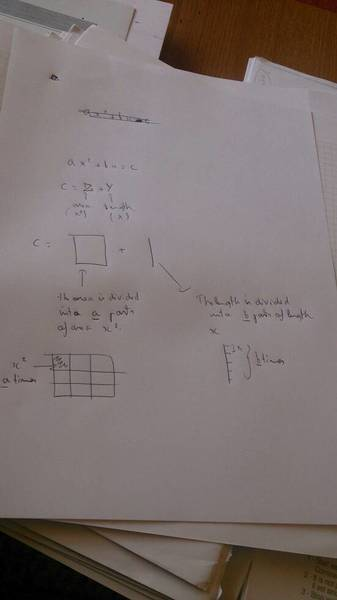# Quadratic equation question

consider the equation:

ax^2+bx=c

imagine that the constant c is divided into to parts:

c=Z+Y

where ax^2= Z and bx=Y
so basically could we say c is part area and part length?? Sorry if the question is not explained better but that's the best way I came up with to describe it.

## Answers and Replies

mfb
Mentor
so basically could we say c is part area and part length??
Are you thinking about physical units?

If x is a length, then a, b and c have to have different units - as an example, a could be a number, b could be a length, and c could be an area:

$$2x^2 + (3m)x = 5m^2$$ has the solution $$x=1m$$

Well i'm considering a and b to be just scalars

NascentOxygen
Staff Emeritus
Science Advisor
Well i'm considering a and b to be just scalars
Then you would be trying to sum an area with a length. This you can't do (nor would you ever want to, anyway).

You are needing a practical example? The cost of putting in a square garden bed comprises 3 expenses:
→ a fixed cost to install a nearby tap,
→ a cost per meter for a brick border around the bed, and
→ a cost per m2 to provide a fixed depth of good soil.

Neither of the co-efficients of the variable is a scalar (without units).

If you are still unconvinced, please explain where/why you believe you would ever want to sum an area and a length?http://imageshack.us/scaled/landing/109/holly1756.gif [Broken]

Last edited by a moderator:
Well I'lll try to explain it with this picture

#### Attachments

•1388147320976.jpg
11.4 KB · Views: 383
NascentOxygen
Staff Emeritus
Science Advisor
Well I'lll explain it with this picture
I'm restricted to using a tablet during this holiday period, and one of its many limitations is that it has no way to display an attachment in a way that I'm able to see the detail of a pic.

Maybe I can set it to show your pic as an inline display? Let's see .....Ah! Now that I can see!

It reveals that you are not wanting to sum an area and a length, just as I foretold.You are actually wanting to sum "pieces" with "pieces". Just as one can sum oranges with oranges, it is equally valid to sum pieces with pieces. The units of your coefficients will not be dimensionless, as we already indicated.For your example, one coefficient will have units of pieces/metre2, the other will have units of pieces/metre. http://physicsforums.bernhardtmediall.netdna-cdn.com/images/icons/icon6.gif [Broken]

Last edited by a moderator:
This is the only way I could explain it, I express myself better with a pen and a paper, it's a shame

rcgldr
Homework Helper
consider the equation:
ax^2+bx=c
imagine that the constant c is divided into to parts:
c=Z+Y
where ax^2= Z and bx=Y
Assumed that x is time, a is the rate of constant acceleration, and b is the initial velocity. Then Y = distance moved related to the initial velocity, and Z is the distance moved related to acceleration. C would also be the initial position at time x = 0.

epenguin
Homework Helper
Gold Member
You can think of it with square bricks. ax2 is a squares of bricks, each side of length a, then bx is a line of b sets of bricks each of length x, or alternatively a rectangle of sides a and x, total number of bricks equals c. You can even work out solution for x whole numbers, even general solution, by moving bricks around. You can envisage a similar thing for cubic equations.

People did sometimes think about them this way. As it is hard to think about quartic and higher equations this way there were at one time many people who said - you mustn't! Such things are 'not physical', not practical, and abstract etc.

No one would say quite that now - but you may well happen to hear some of the same sort of attitude expressed, in tones of great self confidence, by marginally or non-mathematical scientists still today.